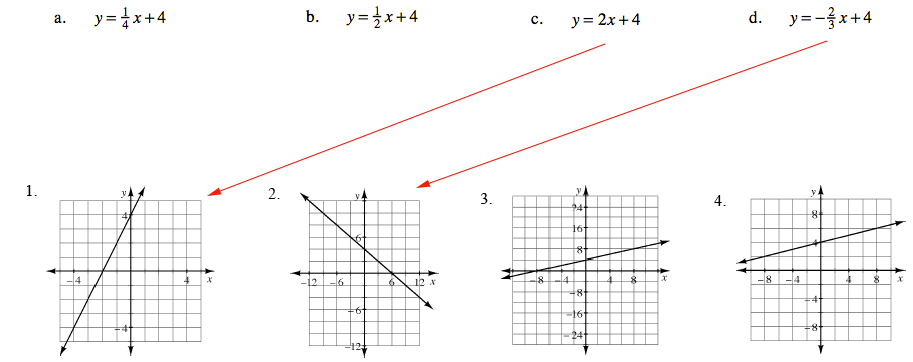Home > CAAC > Chapter 7 > Lesson 7.2.3 > Problem7-76

7-76.

MATCH-A-GRAPH

Match the following graphs with their equations. Pay special attention to the scaling of each set of axes. Explain how you found each match. Homework Help ✎

1. $y=\frac{1}{4}x+4$

1. $y=\frac{1}{2}x+4$

1. $y=2x+4$

1. $y=−\frac{2}{3}x+4$

1.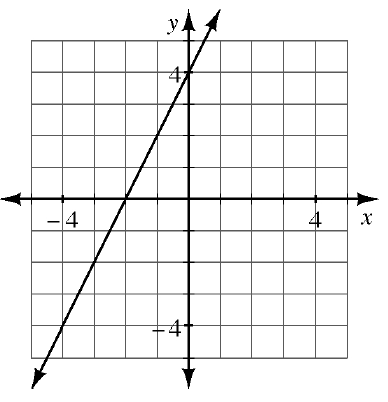1.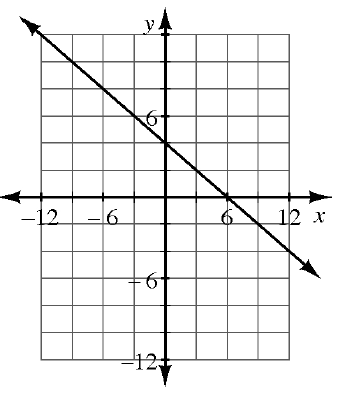1.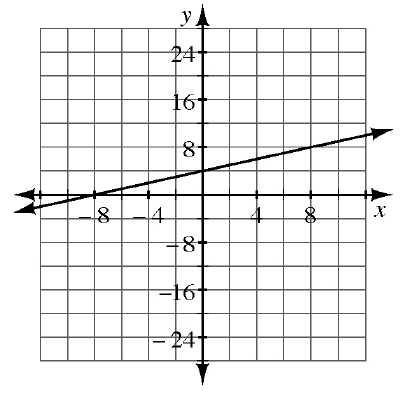1.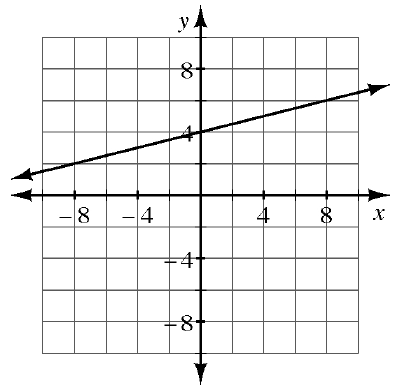Find the corresponding graph for each equation by starting with the $y$-intercept, then use the slope to find the next point.